## General Form for Different Pip Counting Systems

#### Created by Sho Sengoku, 2003

There are good similarities among three different pip counting systems, Douglas Zare's "Half-Crossover Pipcount", Nack Ballard's "Naccel", and my system. Those three systems separate all 26 points of the board in a number of groups, assign a group center, and provide error numbers from the center point to locate any points in a group.

My system, the "Five-Count," is described here.

A detailed explanation for Douglas Zare's "Half-Crossover Pipcount" is available at this link

Nack Ballard's "Naccel" is explained in a GammOnLine article. Note that the "Naccel" shown in GammOnLine is slightly different from the one explained in Backgammon Today Sep. 2001 issue, but is the "bumped" one introduced in the magazine. The "Naccel" I referred in this page is the one with not bumped quads (the 6 point has 6 extra pips.)

To show "summation" operation, I use a "sigma" sign, Σ, as follows:

 15 Σai = Σai = a1 + a2 + a3 + ... + a14 + a15 i=1
I also use these symbols:

 xi = A pip count of a checker located any point. i = 1, 2, ... , 15 P = A total pip count.

### Sho Sengoku's "Five-Count"

xi = 5gi + ei
P = Σ(xi) = 5Σgi + Σei
where
gi = 0, 1, 2, 3, 4, 5 (group)
ei = −2, −1, 0, +1, +2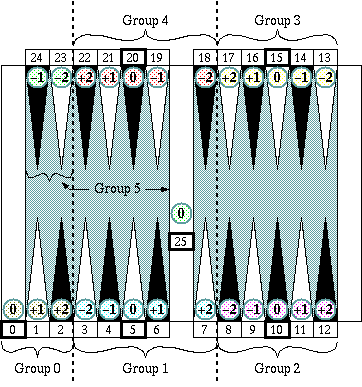### Douglas Zare's "Half-Crossover Pipcount"

xi = 5 + 3hi + ei
P = Σ(xi) = 75 + 3Σhi + Σei
where
hi = −2, −1, 0, 1, 2, 3, 4, 5, 6, 7 (half crossover)
ei = −1, 0, +1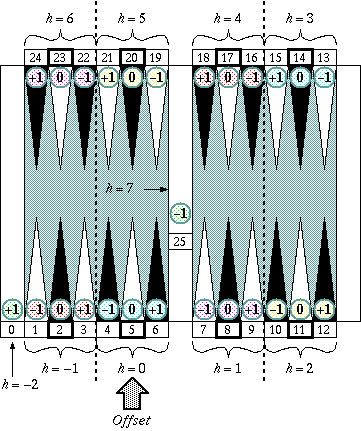Note: the error number of the goal point (or the pocket) is +1 of h = −2.

### Nack Ballard's "Naccel"

xi = 6qi + ei
P = Σ(xi) = 6Σqi + Σei
where
qi = 0, 1, 2, 3, 4 (quad)
ei = +1, +2, +3, +4, +5, +6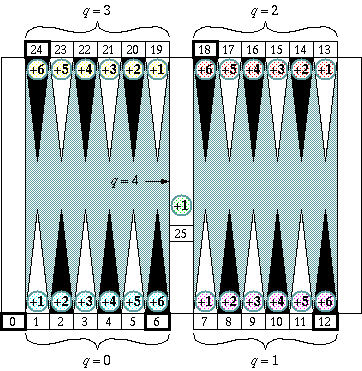Since all ei is positive (+) and Σei often becomes too big to handle for a human player, another breakdown in the Σei part is called for:

Σei = 6s + r
P = 6(Σqi + s) + r
where
s = 0, 1, 2, ..., 15 (squad)
r = 0, 1, 2, ... (remains, usually r < 6)

### Bumped Naccel

xi = 6qi + ei
P = Σ(xi) = 6Σqi + Σei
where
qi = 0, 1, 2, 3, 4 (quad)
ei = 0, +1, +2, +3, +4, +5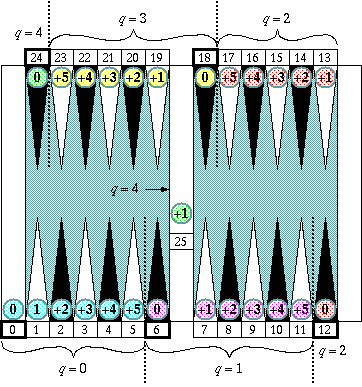### Home-Negative Naccel

xi = 6 + 6qi + ei
P = Σ(xi ) = 90 + 6Σqi + Σei
where
qi = −1, 0, 1, 2, 3
ei = 0, +1, +2, +3, +4, +5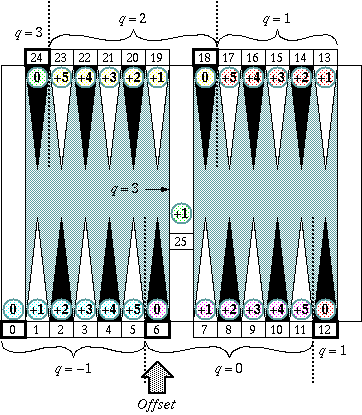### Home-Flop Naccel

xi = 6 + 6qi + ei
P = Σ(xi ) = 90 + 6Σqi + Σei
where
qi = 0, 1, 2, 3
ei = 0, +1, +2, +3, +4, +5 (for qi = 1, 2, 3)
ei = −6, −5, ... , −1, 0, +1, ... , +5 (for qi = 0)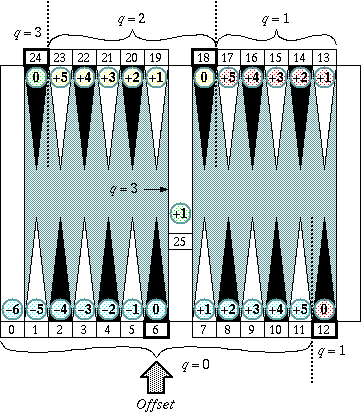Note: the error number of the goal point (or the pocket) is −6.

### General form

 xi = F + Bgi + ei P = Σ(xi ) = 15F + BΣgi + Σei

 B (base) F (offset) gi (group) ei (error) Five-Count 5 0 0, 1, 2, 3, 4, 5 −2, −1, 0, 1, 2 Half-Crossover 3 5 −2, −1, 0, 1, ... , 7 −1, 0, 1 Naccel 6 0 0, 1, 2, 3, 4 1, 2, 3, 4, 5, 6 Bumped Naccel 6 0 0, 1, 2, 3, 4 0, 1, 2, 3, 4, 5 HN Naccel 6 6 −1, 0, 1, 2, 3 0, 1, 2, 3, 4, 5, HF Naccel 6 6 0 1, 2, 3 −6, −5, −4, ... , 4, 5 0, 1, 2, 3, 4, 5

See:  Other articles by Sho Sengoku

See:  Other articles on Pip Counting